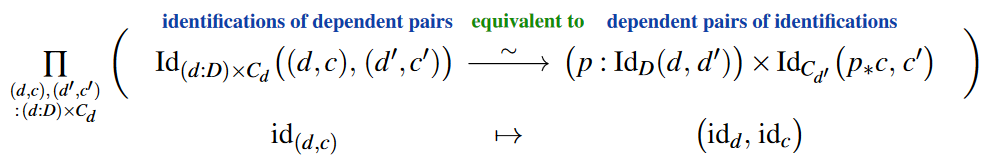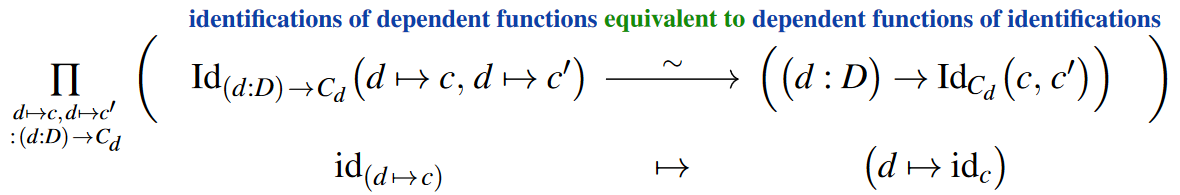Contents

# Contents

## Idea

Where the idea of (non-higher) observational type theory is to equip all type formation rules (notably dependent functions, dependent pairs and inductive constructions) with their dedicated notion of structure preserving definitional equality — namely: component-wise, ie. homo-morphic, hence: “observational” —; the idea of higher observational type theory is to do the same for propositional equality hence for identification types used in homotopy type theory (where types behave like *higher* homomotopy types, whence the qualifier “higher”).

Concretely:

• the “observational” principle for identification of dependent functions is to say that these are dependent functions of identifications of arguments and values (a statement otherwise known as function extensionality),

• the “observational” principle for identifications of dependent pairs is to say that these are dependent pairs of identifications of factors,

and in ordinary univalent homotopy type theory this form of the “structure identity principle” follows as a type equivalence between identification types:The idea of higher observational type theory is to make these and analogous structural characterizations of identification types be part of their definitional inference rules, thus building the structure identity principle right into the rewrite rules of the type theory.

In such a higher observational theory, in particular also the univalence axiom would be a definitional equality and hence would “compute”.

This most desirable property of any homotopy type theory has previously been accomplished only by cubical type theories. However, cubical type theories achieve this only by adding syntax for auxiliary/spurious interval types with rewrite rules which encode technical detail that has no abstract motivation other than making the univalence axiom compute and which one would rather keep out of the syntactic logic and instead relegate to the construction of categorical semantics.

The hope is therefore that higher observational type theory would provide a type system which achieves both:

1. its syntax is as logically clean as that of Martin-Löf dependent type theory equipped with the univalence axiom;

2. its inference rules for identification types make the univalence axiom be a computable function as it is in cubical type theories.

To which extent this hope is being realized would ideally be elucidated by the references below.

## Details

This section does not quite get to the key points across and may need to be largely rewritten from scratch.

We are working in a dependent type theory with judgmental equality.

### Telescopes

telescope types contain lists of iteratively dependent terms

Rules for the empty telescope

$\frac{}{\epsilon\ \mathrm{tel}}$

Rules for telescopes given a telescope and a type

$\frac{\Delta\ \mathrm{tel} \quad \Delta \vdash A \mathrm{type}}{(\Delta,x:A)\ \mathrm{tel}}$

Rules for the empty context in a telescope

$\frac{}{():\epsilon}$

Rules for …

$\frac{\delta:\Delta \quad \Delta \vdash A \mathrm{type} \quad a:A[\delta]}{(\delta,a):(\Delta,x:A)}$

$\frac{\Delta \vdash a:A \quad \delta:\Delta}{a[\delta]:A[\delta]}$

### Identity telescopes

$\frac{\Delta\ \mathrm{tel} \quad \delta:\Delta \quad \delta^{'}:\Delta}{\delta =_\Delta \delta^{'}\ \mathrm{tel}}$

$() =_\epsilon () \equiv \epsilon$

$(\delta,a) =_{\Delta,x:A} (\delta^{'},a^{'}) \equiv \left(\varsigma:\delta =_\Delta \delta^{'}, \alpha:a =_{\Delta.A}^\varsigma a^{'}\right)$

### Dependent identity types

$\frac{\varsigma:\delta =_\Delta \delta^{'} \quad \delta \vdash A\ \mathrm{type} \quad a:A[\delta] \quad a^{'}:A[\delta^{'}]}{a =_{\Delta.A}^\varsigma a^{'}\ \mathrm{type}}$

The identity types in higher observational type theory is defined as

$a =_A a^{'} \equiv a =_{\epsilon.A}^{()} a^{'}$

Computation rules are defined for pair types:

$(s =_{A \times B}^\varsigma t) \equiv (\pi_1(s) =_A^\varsigma \pi_1(t)) \times (\pi_2(s) =_B^\varsigma \pi_2(t))$

Computation rules are defined for function types:

$(f =_{A \to B}^\varsigma g) \equiv \prod_{a:A} \prod_{b:A} \prod_{q:(a =_A b)} (f(a) =_B^\varsigma g(b))$

Computation rules are defined for dependent function types:

$(f =_{\prod_{x:A} B(x)}^\varsigma g) \equiv \prod_{a:A} \prod_{b:A} \prod_{p:(a =_{A}^\varsigma b)} (f(a) =_{B}^{\varsigma,p} g(b))$

Computation rules are defined for dependent pair types:

$(s =_{\sum_{x:A} B(x)}^\varsigma t) \equiv \sum_{p:(\pi_1(s) =_A^\varsigma \pi_1(t))} (\pi_2(s) =_{B}^{\varsigma,p} \pi_2(t))$

### With universes

We are working in a dependent type theory with Tarski-style universes.

The identity types in a universe in higher observational type theory have the following formation rule:

$\frac{A:\mathcal{U} \quad a:\mathcal{T}_\mathcal{U}(A) \quad b:\mathcal{T}_\mathcal{U}(A)}{\mathrm{id}_A(a, b):\mathcal{U}}$

We define a general congruence term called ap

$\frac{x:A \vdash f:B \quad p:\mathrm{id}_A(a, a^{'})}{\mathrm{ap}_{x.f}(p):\mathrm{id}_B(f[a/x], f[a^{'}/x])}$

and the reflexivity terms:

$\frac{a:A}{\mathrm{refl}_{a}:\mathrm{id}_A(a, a)}$

and computation rules for identity functions

$\mathrm{ap}_{x.x}(p) \equiv p$

and for constant functions $y$

$\mathrm{ap}_{x.y}(p) \equiv \mathrm{refl}_{y}$

Thus, ap is a higher dimensional explicit substitution. There are definitional equalities

$\mathrm{ap}_{x.f}(\mathrm{refl}_{a}) \equiv \mathrm{refl}_{f[a/x]}$
$\mathrm{ap}_{y.g}(\mathrm{ap}_{x.f}(p)) \equiv \mathrm{ap}_{x.g[f/y]}(p)$
$\mathrm{ap}_{x.t}(p) \equiv \mathrm{refl}_{t}$

for constant term $t$.

#### Identity types for universes

Let $A \cong_\mathcal{U} B$ be the type of one-to-one correspondences between two terms of a universe $A:\mathcal{U}$ and $B:\mathcal{U}$, and let $\mathrm{id}_\mathcal{U}(A, B)$ be the identity type between two terms of a universe $A:\mathcal{U}$ and $B:\mathcal{U}$. Then there are rules

$\frac{R:A \cong_\mathcal{U} B}{\Delta(R):\mathrm{id}_\mathcal{U}(A, B)} \qquad \frac{P:\mathrm{id}_\mathcal{U}(A, B)}{\nabla(P):A \cong_\mathcal{U} B} \qquad \frac{R:A \cong_\mathcal{U} B}{\nabla(\Delta(R)) \equiv R}$

#### Identity types in universes and singleton contractibility

Given a term of a universe $A:\mathcal{U}$

$\mathrm{id}_{\mathcal{T}_\mathcal{U}(A)} \equiv \pi_1(\nabla(\mathrm{refl}_A))$

with terms representing singleton contractibility.

$\pi_1(\pi_2(\nabla(\mathrm{refl}_A)):\prod_{a:\mathcal{T}_\mathcal{U}(A)} \mathrm{isContr}\left(\sum_{b:\mathcal{T}_\mathcal{U}(A)} \mathrm{id}_{\mathcal{T}_\mathcal{U}(A)}(a, b)\right)$
$\pi_2(\pi_2(\nabla(\mathrm{refl}_A))):\prod_{b:\mathcal{T}_\mathcal{U}(A)} \mathrm{isContr}\left(\sum_{a:\mathcal{T}_\mathcal{U}(A)} \mathrm{id}_{\mathcal{T}_\mathcal{U}(A)}(a, b)\right)$

#### Dependent identity types in universes

Given a term of a universe $A:\mathcal{U}$, a judgment $z:\mathcal{T}_\mathcal{U}(A) \vdash B:\mathcal{U}$, terms $x:\mathcal{T}_\mathcal{U}(A)$ and $y:\mathcal{T}_\mathcal{U}(A)$, and an identity $p:\mathrm{id}_{\mathcal{T}_\mathcal{U}(A)}(x,y)$, we have

$\mathrm{ap}_{z.B}(p):\mathrm{id}_\mathcal{U}(B(x),B(y))$

and

$(u,v):\mathcal{T}_\mathcal{U}(B(x)) \times \mathcal{T}_\mathcal{U}(B(y)) \vdash \pi_1(\nabla(\mathrm{ap}_{z.B}(p)))(u,v):\mathcal{U}$

We could define a dependent identity type as

$\mathrm{id}_{\mathcal{T}_\mathcal{U}(z.B)}^{p}(u, v) \coloneqq \pi_1(\nabla(\mathrm{ap}_{z.B}(p)))(u, v)$

There is a rule

$\frac{A:\mathcal{U} \quad z:\mathcal{T}_\mathcal{U}(A) \vdash B:\mathcal{U} \quad a:\mathcal{T}_\mathcal{U}(A)}{\mathrm{id}_{\mathcal{T}_\mathcal{U}(z.B)}^{\refl_{a}}(u, v) \equiv \mathrm{id}_{\mathcal{T}_\mathcal{U}(B[a/z])}(u, v)}$

and for constant families $B:\mathcal{U}$

$\mathrm{id}_{\mathcal{T}_\mathcal{U}(z.B)}^{p}(u, v) \equiv \mathrm{id}_{\mathcal{T}_\mathcal{U}(B)}(u, v)$